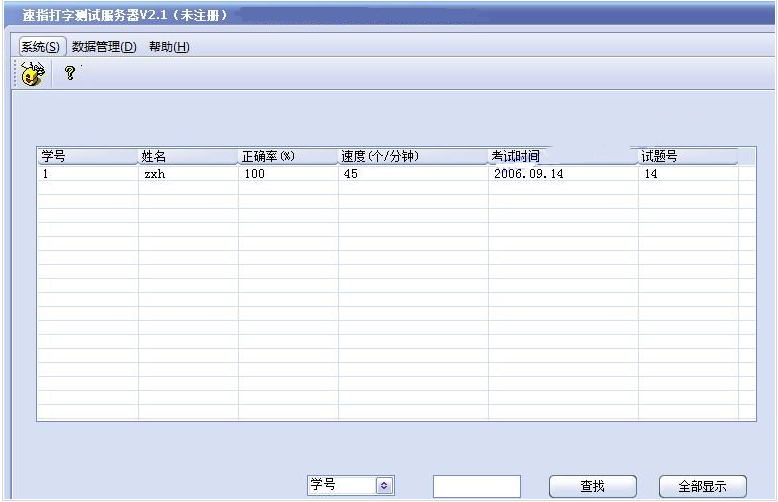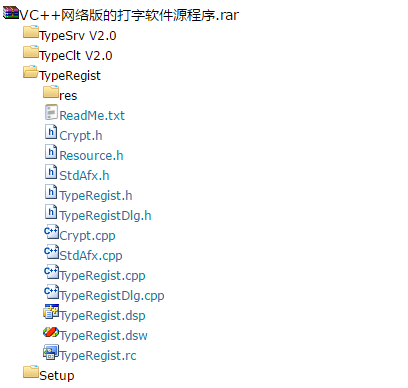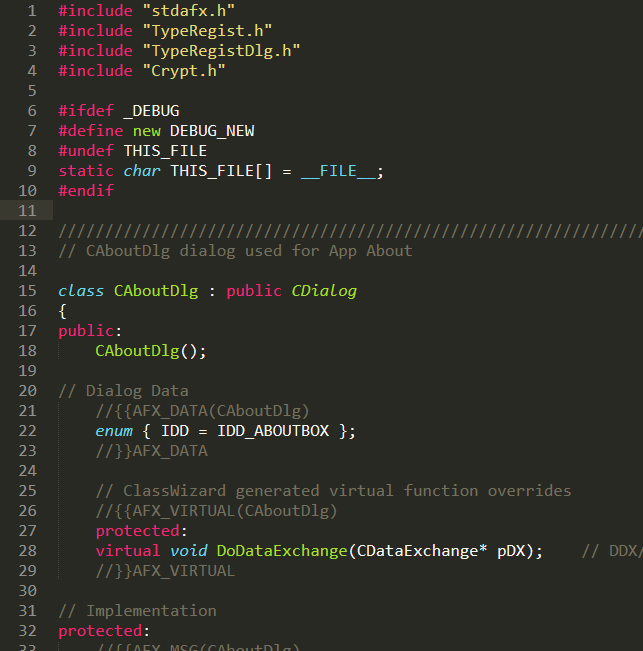2017-07-19 17:23:43 liuyuxin123 阅读数 5994
• ###### 深入浅出C++程序设计(基础篇）视频教学

C/C++都是比较早的、也是非常经典的编程语言，至今仍然非常流行。本教程结合个人超过10年的C/C++的使用经验，调试完成整套深入浅出C++程序设计教程，包括基础篇、面向对象篇、C++STL标准模板库，每篇都适合不同层次的C/C++爱好者学习与使用。

12548 人正在学习 去看看 李建祥

``````第一组
1 2 +

1 0 /

1 0 XOR``````

``````第一组
3

Divided by zero!

Invalid operator!``````

``````//Data:June 19, 2017
//Writen by Yuxin Liu
#include <iostream>
using namespace std;
int main()
{
int a = { 0, 0 };//Initialization
char b = ' ';
//输入
for (int i = 0; i < 2; i++)
{
cin >> a[i];
}
cin >> b;
//switch语句匹配相关运算符
switch (b)
{
case '+' :
cout << a + a;
break;
case '-' :
cout << a - a;
break;
case '*' :
cout << a * a;
break;
case '/' :
if (a==0)
{
cout << "Divided by zero!";
break;
}
else
{
cout << a / a;
break;
}
default:
cout << "Invalid operator !";

}
return 0;
}``````

2014-04-06 20:14:25 guodongxiaren 阅读数 2987
• ###### 深入浅出C++程序设计(基础篇）视频教学

C/C++都是比较早的、也是非常经典的编程语言，至今仍然非常流行。本教程结合个人超过10年的C/C++的使用经验，调试完成整套深入浅出C++程序设计教程，包括基础篇、面向对象篇、C++STL标准模板库，每篇都适合不同层次的C/C++爱好者学习与使用。

12548 人正在学习 去看看 李建祥

—————————————————————————————————————————

我们经常会遇到使用C/C++语言编写一个菜单系统的情况。在控制台窗口打印一个有好多选项的菜单，然后提示你通过输入对应的编号来完成相应的操作。

这不，我们数据结构课，老师也是叫我们把一种数据结构的所有操作输出到一个菜单里，通过输入序号来测试每个操作的功能。老师给出了我们大体上框架的代码，但是老师使用的是switch来执行菜单，代码较多重复，相当麻烦。于是我就使用函数指针数组来替代实现了相同的功能。

——————————————————————————————————————————

# 本文的写作背景```cin>>choose;//整型
if(choose>0&&choose<14)
{
system("cls");//VC的清屏函数，gcc不适用
displayCurrentObject(sq);//打印当前顺序表的每个元素
}
switch(choose)
{
case 1:ex3_1_1(sq,continueYesNo);break;
case 2:ex3_1_2(sq,continueYesNo);break;
case 3:ex3_1_3(sq,continueYesNo);break;
case 4:ex3_1_4(sq,continueYesNo);break;
case 5:ex3_1_5(sq,continueYesNo);break;
case 6:ex3_1_6(sq,continueYesNo);break;
case 7:ex3_1_7(sq,continueYesNo);break;
case 8:ex3_1_8(sq,continueYesNo);break;
case 9:ex3_1_9(sq,continueYesNo);break;
case 10:ex3_1_10(sq,continueYesNo);break;
case 11:ex3_1_11(sq,continueYesNo);break;
case 12:ex3_1_12(sq,continueYesNo);break;
case 13:ex3_1_13(sq,continueYesNo);break;

default:    cout<<"\n  你选择了结束"<<endl<<endl;
return;
}
//判断是否继续操作
if(continueYesNo=='N'||continueYesNo=='n')
break;```

```//ex3_1_Test.h单独的一个头文件
//测试：判断顺序表是否为空
template <typename ElemType>
void ex3_1_2(MySqList<ElemType>& sq,char& continueYesNo)
{
cout<<" ***********\4判断顺序表是否为空\4**********"<<endl<<endl;
if(sq.isEmpty())
cout<<"当前顺序表为空"<<endl;
else
{
cout<<"当前顺序表不为空"<<endl;
cout<<sq;
}
cout<<" *****************************************"<<endl<<endl;
cout<<" 还继续吗(Y.继续\tN.结束)?";
cin>>continueYesNo;
}```

# 函数指针数组替代switch

```//main函数所在的cpp文件中
void (*test)(MySqList<int>&,char&)={ex3_1_1,ex3_1_2,ex3_1_3,ex3_1_4,
ex3_1_5,ex3_1_6,ex3_1_7,ex3_1_8,
ex3_1_9,ex3_1_10,ex3_1_11,ex3_1_12,
ex3_1_13};```

```cin>>choose;
if(choose>0&&choose<14)
{
system("cls");
displayCurrentObject(sq);
}
test[choose-1](sq,continueYesNo);
if(continueYesNo=='N'||continueYesNo=='n')
break;```

`void (*test[])(形参列表)={……};`

# 小讲解函数指针及其数组

```//功能：判断两整数是否相等
bool equal(int a,int b)
{
if(a==b)
return true;
else
return false;
}
//功能：判断一个整数是否大于另一个整数
bool great(int a,int b)
{
if(a>b)
return true;
else
return false;
}
//功能：判断一个整数是否小于另一个整数
bool less(int a,int b)
{
if(a<b)
return true;
else
return false;
}```

```bool fun(int a,int b,bool(*compare)(int,int))
{
return (*compare)(a,b);
}```

bool(*)(int,int) 是参数的类型，compare是该类型的变量名，也是起什么名字无所谓的。再看指针数组比如：

`bool(*fun)(int,int)={equal,great,less};`

```bool(*fun2)(int,int);
fun2 = equal;
fun2 = great;
fun2 = less;```

```//这是等效的操作
fun2 = equal;
fun2 = &equal;
//注意！这里的&是取地址符号，而非引用符号```

2019-10-19 16:04:29 weixin_45287871 阅读数 61
• ###### 深入浅出C++程序设计(基础篇）视频教学

C/C++都是比较早的、也是非常经典的编程语言，至今仍然非常流行。本教程结合个人超过10年的C/C++的使用经验，调试完成整套深入浅出C++程序设计教程，包括基础篇、面向对象篇、C++STL标准模板库，每篇都适合不同层次的C/C++爱好者学习与使用。

12548 人正在学习 去看看 李建祥C++网络版的打字软件源程序，程序会连接远程数据库获取打字信息，并包括有打字练习、网上考试模块，本程序编写时要用到很多网络知识、数据库知识以及字符处理功能等。 提示：编译完成后要将SkinPlusPlusDLL.dll拷贝至Debug目录中，TypeData.mdb放入Debug\Data中。2019-05-30 17:00:36 weixin_41986518 阅读数 1008
• ###### 深入浅出C++程序设计(基础篇）视频教学

C/C++都是比较早的、也是非常经典的编程语言，至今仍然非常流行。本教程结合个人超过10年的C/C++的使用经验，调试完成整套深入浅出C++程序设计教程，包括基础篇、面向对象篇、C++STL标准模板库，每篇都适合不同层次的C/C++爱好者学习与使用。

12548 人正在学习 去看看 李建祥2016-11-23 19:41:36 longjingwen12345 阅读数 1453
• ###### 深入浅出C++程序设计(基础篇）视频教学

C/C++都是比较早的、也是非常经典的编程语言，至今仍然非常流行。本教程结合个人超过10年的C/C++的使用经验，调试完成整套深入浅出C++程序设计教程，包括基础篇、面向对象篇、C++STL标准模板库，每篇都适合不同层次的C/C++爱好者学习与使用。

12548 人正在学习 去看看 李建祥

C++实现双向循环链表，包括链表的创建，插入，删除，查询等基本操作，并带有操作菜单，见底部效果图

``````// 双向循环链表3.cpp : 定义控制台应用程序的入口点。
//

#include "stdafx.h"
#include <iostream>
using namespace std;

//每个链表对象的结构
typedef struct node {
int data;
struct node *pre;
struct node *next;
}Dnode;

//创建一个带头结点的双向循环链表
{
Dnode *p, *q;
int x;
int i = 1;//结点位置
q = (Dnode *)malloc(sizeof(Dnode));
if (q == NULL)
{
cout << "内存分配失败！" << endl;

}
q->data = 0;
q->pre = q;
q->next = q;

cout << "请输入第" << i << "个结点值：";
cin >> x;
cout << endl;
while (x != 0)
{
p = (Dnode *)malloc(sizeof(Dnode));
if (p == NULL)
{
cout << "内存分配失败！" << endl;

}
p->data = x;
p->pre = q;
q->next = p;
q = p;
i++;
cout << "请输入第" << i << "个结点值：";
cin >> x;
cout << endl;
}
}

//遍历链表
{
p = L->next;
cout << p->data <<"  ";
p = p->next;
}
cout << endl;
}

//返回链表的大小
int length(Dnode *L)
{

int sum = 0;
sum++;
p = p->next;
}
return sum;
}
//获取第i个结点前的位置
Dnode * getPosition(Dnode *L, int i)
{

Dnode *head = L, *p = L->next;

int j = 1;
while (p != head&&j<(i - 1))
{
p = p->next;
j++;
}
return p;

}

//向链表第i个位置，插入数据为e的结点
int insertElemToLinkList(Dnode *L, int i, int e)
{

int sum = length(L);
if (i<1 || i>(sum + 1))
{
cout << "请输入正确的插入位置" << endl;
return 0;
}

q = (Dnode *)malloc(sizeof(Dnode));
if (q == NULL)
{
cout << "内存分配失败!" << endl;
return 0;
}
//在链表头部插入
if (i == 1)
{
q->data = e;
return 1;
}

else {
p = getPosition(L, i);
q->data = e;
q->next = p->next;
q->pre = p;
p->next->pre = q;
p->next = q;
return 1;

}
return 0;

}
//删除第i个结点
{

p = L;
int j = 1;
int sum = length(L);
if (i<1 || i>sum)
{
cout << "删除位置超出聊表的范围!" << endl;
return ;
}

while (j <= i)
{
p = p->next;
j++;
}
int k = p->data;
p->pre->next = p->next;
p->next->pre = p->pre;
free(p);

}

//查找链表中是否有某个值
Dnode * search(Dnode *L, int e)
{

p = L->next;
while (p != head&&p->data != e)
{
p = p->next;
}
{
return p;
}
else
{
return NULL;
}
}

void main()
{
Dnode *L = NULL;
//让程序成菜单形式，不会退出
while (true)
{
int m = 0;//菜单项
int i, e;
cout << endl;
cout << "    ***********************************************************" << endl;
cout << "    *                                                         *" << endl;
cout << "    *     1、创建链表                                         *"<< endl;
cout << "    *     2、遍历链表                                         *" << endl;
cout << "    *     2、求链表长度                                       *" << endl;
cout << "    *     4、向链表的第i个位置插入新结点（i>=1）              *" << endl;
cout << "    *     5、删除链表中的的第i个值（i>=1）                    *"<< endl;
cout << "    *     6、查询链表中是否有key的结点                        *"<< endl;
cout << "    *                                                         *" << endl;
cout << "    ***********************************************************" << endl;
cout << "请输入菜单序号,执行相应操作：";
cin >> m;
cout << endl;
if (L == NULL&&m!=1)
{
cout <<"请先初始化链表，再执行其它相关操作"<<endl ;
m = 1;
}
switch (m)
{
case 1://创建链表
break;
case 2://遍历链表

break;
case 3://求链表长度
cout << "链表的长度为：" << length(L) << endl;
break;
case 4://向链表的第i个位置插入新结点（i>=1）
cout << "插入新节点，请输入待插入的值和位置：" << endl;
cin >> e >> i;
cout << endl;
cout << "新链表的所有元素输出如下：" << endl;
break;
case 5://删除链表中的的第i个值（i>=1）
cout << "删除聊表中的第i个元素，请输入要删除的位置（位置从1开始计数）：";
cin >> i;
cout << endl;
cout << "新链表的所有元素输出如下：" << endl;
break;
case 6://查询链表中是否有key的结点
cout << "查询链表中是否有某个数，请输入需要查询的key:";
cin >> e;
cout << endl;
Dnode *m = search(L, e);
if (m != NULL)
{
cout << "链表中存在" << e <<endl;
}
break;
}
}
}

``````